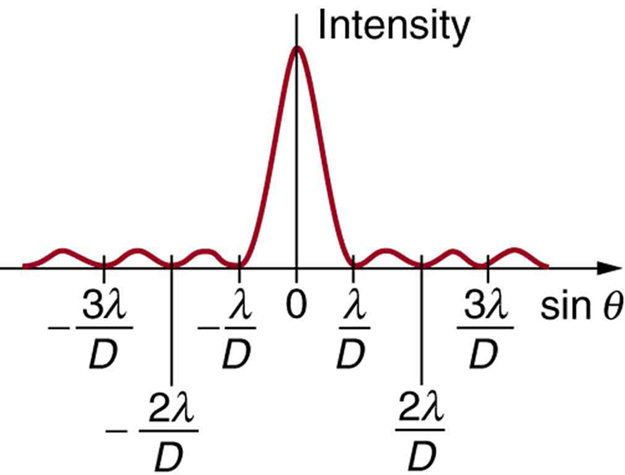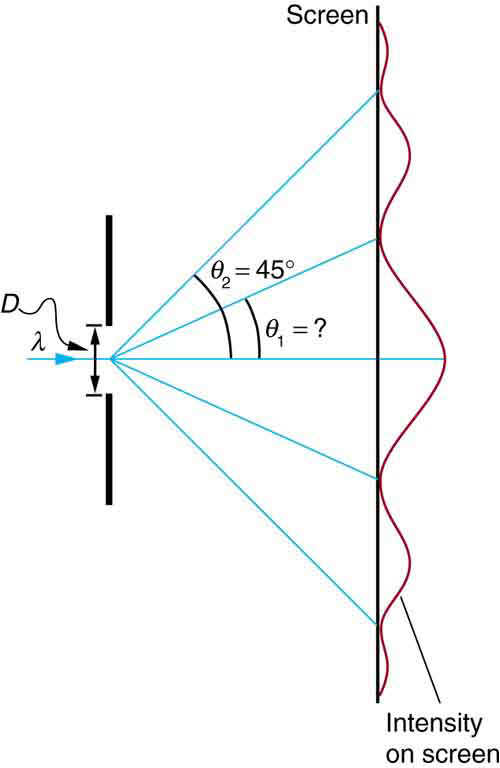27.5 Single slit diffraction  (Page 2/4)

 Page 2 / 4A graph of single slit diffraction intensity showing the central maximum to be wider and much more intense than those to the sides. In fact the central maximum is six times higher than shown here.

Thus, to obtain destructive interference for a single slit    ,

$D\text{sin}\phantom{\rule{0.25em}{0ex}}\theta =\mathrm{m\lambda },\phantom{\rule{0.25em}{0ex}}\text{for}\phantom{\rule{0.25em}{0ex}}m=\text{1,}\phantom{\rule{0.25em}{0ex}}\text{–1,}\phantom{\rule{0.25em}{0ex}}\text{2,}\phantom{\rule{0.25em}{0ex}}\text{–2,}\phantom{\rule{0.25em}{0ex}}\text{3,}\phantom{\rule{0.25em}{0ex}}\dots \phantom{\rule{0.25em}{0ex}}\text{(destructive),}$

where $D$ is the slit width, $\lambda$ is the light’s wavelength, $\theta$ is the angle relative to the original direction of the light, and $m$ is the order of the minimum. [link] shows a graph of intensity for single slit interference, and it is apparent that the maxima on either side of the central maximum are much less intense and not as wide. This is consistent with the illustration in [link] (b).

Calculating single slit diffraction

Visible light of wavelength 550 nm falls on a single slit and produces its second diffraction minimum at an angle of $\text{45.0º}$ relative to the incident direction of the light. (a) What is the width of the slit? (b) At what angle is the first minimum produced?A graph of the single slit diffraction pattern is analyzed in this example.

Strategy

From the given information, and assuming the screen is far away from the slit, we can use the equation $D\text{sin}\phantom{\rule{0.25em}{0ex}}\theta =\text{mλ}$ first to find $D$ , and again to find the angle for the first minimum ${\theta }_{1}$ .

Solution for (a)

We are given that $\lambda =\text{550 nm}$ , $m=2$ , and ${\theta }_{2}=\text{45.0º}$ . Solving the equation $D\phantom{\rule{0.25em}{0ex}}\text{sin}\phantom{\rule{0.25em}{0ex}}\theta =\text{mλ}$ for $D$ and substituting known values gives

$\begin{array}{lll}D& =& \frac{\mathrm{m\lambda }}{\phantom{\rule{0.25em}{0ex}}\text{sin}\phantom{\rule{0.25em}{0ex}}{\theta }_{2}}=\frac{2\left(\text{550 nm}\right)}{\text{sin 45.0º}}\\ & =& \frac{\text{1100}×{\text{10}}^{-9}}{0.707}\\ & =& 1.56×{\text{10}}^{-6}.\end{array}$

Solution for (b)

Solving the equation $D\phantom{\rule{0.25em}{0ex}}\text{sin}\phantom{\rule{0.25em}{0ex}}\theta =\text{mλ}$ for $\text{sin}\phantom{\rule{0.25em}{0ex}}{\theta }_{1}$ and substituting the known values gives

$\text{sin}\phantom{\rule{0.25em}{0ex}}{\theta }_{1}=\frac{\mathrm{m\lambda }}{D}=\frac{1\left(\text{550}×{\text{10}}^{-9}\phantom{\rule{0.25em}{0ex}}\text{m}\right)}{1\text{.}\text{56}×{\text{10}}^{-6}\phantom{\rule{0.25em}{0ex}}\text{m}}\text{.}$

Thus the angle ${\theta }_{1}$ is

${\theta }_{1}={\text{sin}}^{-1}\phantom{\rule{0.25em}{0ex}}\text{0.354}=\text{20.7º.}$

Discussion

We see that the slit is narrow (it is only a few times greater than the wavelength of light). This is consistent with the fact that light must interact with an object comparable in size to its wavelength in order to exhibit significant wave effects such as this single slit diffraction pattern. We also see that the central maximum extends $\text{20.7º}$ on either side of the original beam, for a width of about $\text{41º}\phantom{\rule{0.25em}{0ex}}$ . The angle between the first and second minima is only about $\text{24º}\phantom{\rule{0.25em}{0ex}}\left(\text{45.0º}-20.7º\right)$ . Thus the second maximum is only about half as wide as the central maximum.

Section summary

• A single slit produces an interference pattern characterized by a broad central maximum with narrower and dimmer maxima to the sides.
• There is destructive interference for a single slit when $D\phantom{\rule{0.25em}{0ex}}\text{sin}\phantom{\rule{0.25em}{0ex}}\theta =\text{mλ},\phantom{\rule{0.25em}{0ex}}\text{(for}\phantom{\rule{0.25em}{0ex}}m=\text{1,}\phantom{\rule{0.25em}{0ex}}\text{–1,}\phantom{\rule{0.25em}{0ex}}\text{2,}\phantom{\rule{0.25em}{0ex}}\text{–2,}\phantom{\rule{0.25em}{0ex}}\text{3,}\phantom{\rule{0.25em}{0ex}}\dots \right)$ , where $D$ is the slit width, $\lambda$ is the light’s wavelength, $\theta$ is the angle relative to the original direction of the light, and $m$ is the order of the minimum. Note that there is no $m=0$ minimum.

Conceptual questions

As the width of the slit producing a single-slit diffraction pattern is reduced, how will the diffraction pattern produced change?

Problems&Exercises

(a) At what angle is the first minimum for 550-nm light falling on a single slit of width $1\text{.}\text{00}\phantom{\rule{0.25em}{0ex}}\text{μm}$ ? (b) Will there be a second minimum?

(a) $\text{33}\text{.}4º$

(b) No

(a) Calculate the angle at which a $2\text{.}\text{00}\text{-μm}$ -wide slit produces its first minimum for 410-nm violet light. (b) Where is the first minimum for 700-nm red light?

the definition of photon
8kg of a hot liquid initial T is 90°© is missed with another liquid 3kg at 20° calculate e équilibrium T
8kg of a hot liquid initial T is 90°© is missed with another liquid 3kg at 20° calculate e équilibrium T
Balki
Bright
what are the products when acid and base mixed?
Austin
salt and water
what work done
work done is the product of force and distance moved in the direction of force
Work done = force (F) * distance (D)
abdulsalam
what is resounance
Abdul
y
Tracy
explain the three laws of isaac Newton with the reference
1st law ; a body will continue to stay at a state of rest or continue to move at a uniform motion on a straight line unless an external force is been acted upon
Austine
3rd law; in every action there is an equal or opposite reaction
Austine
2nd law: F=ma
Austine
why am i not having access to the Link in your exemples /figures ?
what is circut
newtons law of motion
hasiya
First law:In an inertial frame of reference, an object either remains at rest or continues to move at a constant velocity, unless acted upon by a force.
Manan
is the ability to do work
Energy
Nwany
u from
Hejreen
any body online hain
Hejreen
ability to do work is energy
what is energy
energy is ability of the capacity to doing work
shafiu
what is vector
A quantity that has both magnitude and direction
Donaldo
can a body with out mass float in space
mosco
Is the quantity that has both magnitude and direction
Amoah
Yes it can float in space,e.g.polyethene has no mass that's why it can float in space
Amoah
that's my suggestion,any other explanation can be given also,thanks
Amoah
A charge of 1.6*10^-6C is placed in a uniform electric field in a density 2*5^10Nc^-1, what is the magnitude of the electric force exerted on the charge?
what's phenomena
Phenomena is an observable fact or event.
Love
Prove that 1/d+1/v=1/f
What interference
What is a polarized light called?
Moyinoluwa
what is a half life
the time taken for a radioactive element to decay by half of its original mass
ken
what is radioactive element
mohammed
Half of the total time required by a radioactive nuclear atom to totally disintegrate
Justice
radioactive elements are those with unstable nuclei(ie have protons more than neutrons, or neutrons more than protons
Justice
in other words, the radioactive atom or elements have unequal number of protons to neutrons.
Justice
state the laws of refraction
Fabian
state laws of reflection
Fabian
Why does a bicycle rider bends towards the corner when is turning?
Mac
When do we say that the stone thrown vertically up wards accelerate negatively?
Mac
Give two importance of insulator placed between plates of a capacitor.
Mac
Macho had a shoe with a big sole moving in mudy Road, shanitah had a shoe with a small sole. Give reasons for those two cases.
Mac
when was the name taken from
retardation of a car
Biola
when was the name retardation taken
Biola
did you mean a motion with velocity decreases uniformly by the time? then, the vector acceleration is opposite direction with vector velocity
Sphere
what's velocity
mosco
Velocity is the rate of change of displacement
DivyaByByByByBy David MartinBy